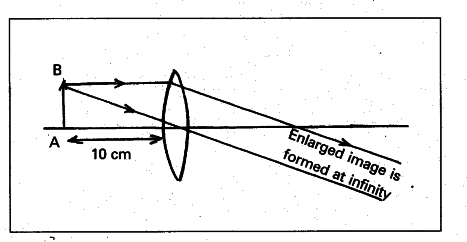# You are given a convex lens of the focal length 10 cm

You are given a convex lens of focal length 10 cm. Where would you place an object to get a real inverted and highly enlarged image of the object ? Draw a ray diagram.

If an object is placed at the focus of the lens it forms real, inverted and highly enlarged image. Thus, the distance of the object from the optical centre of the lens is equal to the focal length of the lens = 10 cm The ray diagram is as shown